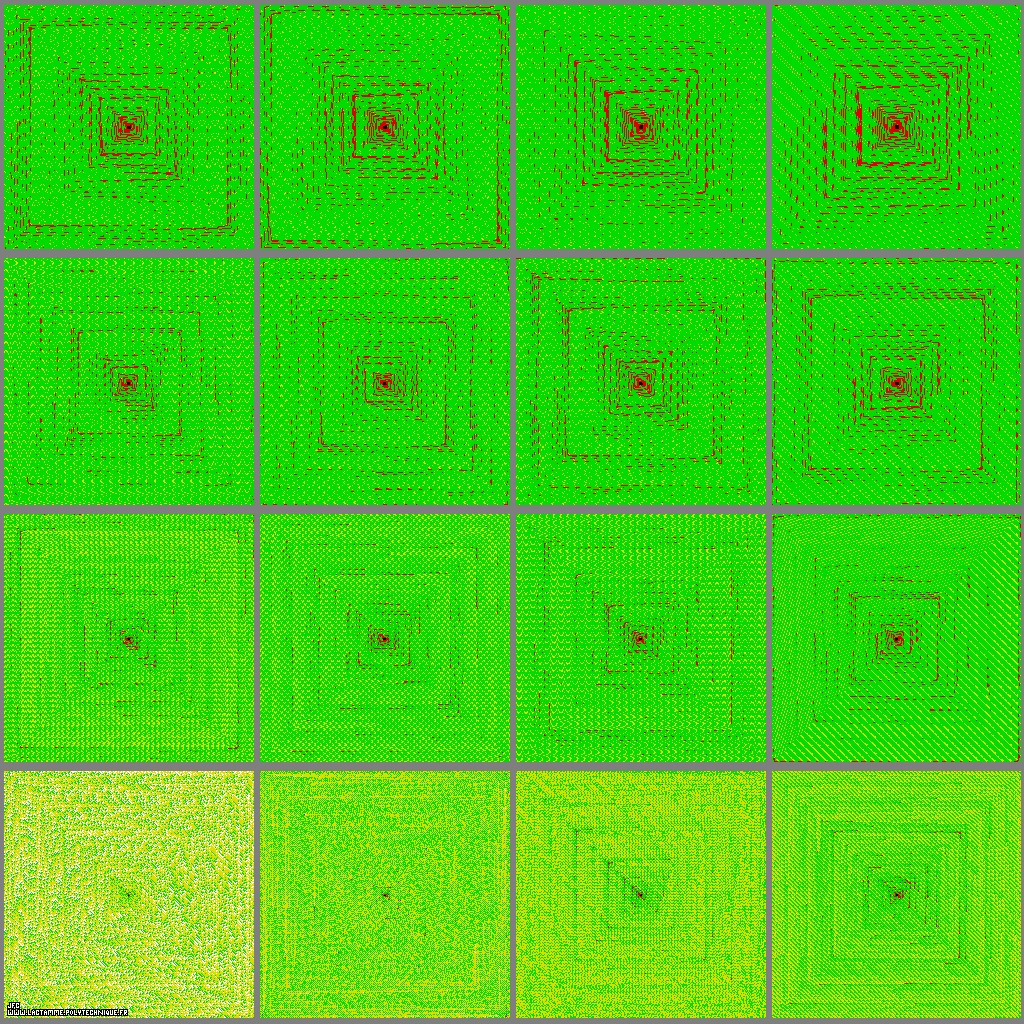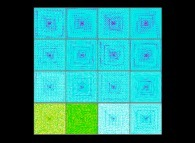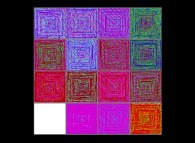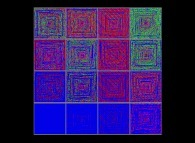The additive persistence of the 65536 first integer numbers for the bases 2 -lower left- to 17 -upper right- [La persistance additive des 65536 premiers nombres entiers pour les bases 2 -en bas et à gauche- à 17 -en haut et à droite-].

• Starting from the origin of the coordinates (at the center of the picture), one follows a square spiral-like path and numbers each integer point encountered (1, 2, 3,...):

```                    5----4----3
|         |    .
|         |    .
6    1----2    .
|              |
|              |
7----8----9----10
```

• Then one displays the N-th point with the false color f(PA(N,B)) where:
```                    PA(N,B) = the additive persistence of N for the base B,
f(...)  = an arbitrary ascending function
```
Let's define PA(N,B) with an obvious example:
```                    B = 10
2      1      0
N = 856 (= 8xB  + 5xB  + 6xB )
```
Then the following sequence is computed:

## ``` 856 ---> (8+5+6) = 19 ---> (1+9) = 10 ---> (1+0) = 1 1 2 3 ```

It takes three (3) steps to reach a one digit number. Then:
```                    PA(856,10) = 3
```

• More information on this subject is available in the august 2013 issue of PLS (Pour La Science) with the Jean-Paul Delahaye's paper La persistance des nombres.

In this set of 4x4 pictures -with global renormalization- only the maximum of the sixteen maxima of PA(N,B) (B={2,3,...,16,17}) is displayed as a white point (in fact too small to be seen).

See a related picture:See some pictures related to the multiplicative persistence:(CMAP28 WWW site: this page was created on 05/31/2013 and last updated on 04/19/2019 14:56:36 -CEST-)

[See all related pictures (including this one) [Voir toutes les images associées (incluant celle-ci)]]

[Go back to AVirtualMachineForExploringSpaceTimeAndBeyond [Retour à AVirtualMachineForExploringSpaceTimeAndBeyond]]
[The Y2K bug [Le bug de l'an 2000]]

[Site Map, Help and Search [Plan du Site, Aide et Recherche]]
[Mail [Courrier]]
[About Pictures and Animations [A Propos des Images et des Animations]]